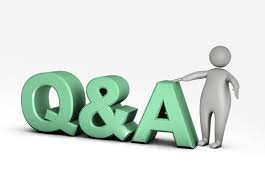# SCC Education

## Profit and loss (Questions)Profit and loss (Questions)
Section A
(i) The discount is always on the _______.
(A) Marked Price (B) Cost Price (C) Selling Price (D) Interest
(ii) If M.P. = Rs.140, S.P. = Rs 105, then Discount = _______.
(A) Rs 245 (B) Rs 25 (C) Rs 30 (D) Rs 35
(iii) ______ = Marked Price – Discount.
(A) Cost Price (B) Selling Price (C) List Price (D) Market price
(iv) The tax added to the value of the product is called ______ Tax.
(A) Sales Tax (B) VAT (C) Excise Tax (D) Service Tax
(v) If the S.P. of an article is Rs 240 and the discount given on it is ` 28, then the M.P. is _______.
(A) Rs 212 (B) Rs 228 (C) Rs 268 (D) Rs 258
Section B

1. A bicycle marked at Rs 1,500 is sold for Rs 1,350. What is the percentage of discount?

2. The list price of a frock is Rs 220. A discount of 20% on sales is announced. What is the amount of discount on it and its selling price?

3. An almirah is sold at Rs 5,225 after allowing a discount of 5%. Find its marked price.

4. A shopkeeper allows a discount of 10% to his customers and still gains 20%. Find the marked price of an article which costs Rs 450 to the shopkeeper.

5. A dealer allows a discount of 10% and still gains 10%. What is the cost price of the book which is marked at ` 220?

6. A television set was sold for Rs 14,400 after giving successive discounts of 10% and 20% respectively. What was the marked price?

7. A trader buys an article for Rs 1,200 and marks it 30% above the C.P. He then sells it after allowing a discount of 20%. Find the S.P. and profit percent.

8. Vinod purchased musical instruments for Rs 12,000. If the rate of sales tax is 8%, find the sales tax and the total amount paid by him.

9. A refrigerator is purchased for Rs 14,355, including sales tax. If the actual cost price of the refrigerator is ` 13,050, find the rate of sales tax

10. Priya bought a suitcase for Rs 2,730. The VAT for this item is 5%. What was the price of the suitcase before VAT was added? Also state how much is the VAT?

11. The price marked on a book is Rs 450. The shopkeeper gives 20% discount on it in a book exhibition. What is the Selling Price?

12 . A television set was sold for Rs 5,760 after giving successive discounts of 10% and  20% respectively. What was the Marked Price?

13. Sekar bought a computer for Rs 38,000 and a printer for Rs  8,000. If the rate of sales tax is 7% for these items, fi nd the price he has to pay to buy these two items.

14. The selling price with VAT, on a cooking range is Rs 19,610. If the VAT is 6%, what is the original price of the cooking range?

15. The sales tax on a refrigerator at the rate of 9% is Rs 1,170. Find the actual sale price.

16. A T.V. with marked price Rs 11,500 is sold at 10% discount. Due to festival season, the shop keeper allows a further discount of 5%. Find the net selling price of the T.V.

17. A person pays Rs 2,800 for a cooler listed at Rs 3,500. Find the discount percent offered.

18. Deepa purchased 15 shirts at the rate of Rs 1,200 each and sold them at a profit of 5%. If the customer has to pay sales tax at the rate of 4%, how much will one shirt cost to the customer?

19. Which is a better offer? Two successive discounts of 20% and 5% or a single discount of 25%. Give appropriate reasons.

20.What price should Suneeta mark on a sari which costs her Rs 2200 so as to gain 26 % after allowing a discount of 12 %?

21. A trader marks his goods 40% above the cost price. He sells them at a discount of 5%. What is his loss or gain percentage?

22. Richard got a discount of 10% on the suit he bought. The marked price was Rs 5,000 for the suit. If he had to pay sales tax of 10% on the price at which he bought, how much did he pay?

formula-sheet
properties-of-parallel-lines
parallelograms properties
volume-and-area-of-cone-and-pyramid
profit-and-loss-questions
compound-interest
volumes-surface-and-area
percentage-and-its-application